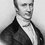# help!!!!!!!!!!

Please give a hint how to solve this algebra problem:- If p and q are roots of the equation:- $x^{2}-a(x-1)+b$ Then find out the value of:- $\frac{1}{p^{2}-ap}+\frac{1}{q^{2}-bq}+\frac{2}{a+b}$Note by Aman Sharma
6 years, 9 months ago

This discussion board is a place to discuss our Daily Challenges and the math and science related to those challenges. Explanations are more than just a solution — they should explain the steps and thinking strategies that you used to obtain the solution. Comments should further the discussion of math and science.

When posting on Brilliant:

• Use the emojis to react to an explanation, whether you're congratulating a job well done , or just really confused .
• Ask specific questions about the challenge or the steps in somebody's explanation. Well-posed questions can add a lot to the discussion, but posting "I don't understand!" doesn't help anyone.
• Try to contribute something new to the discussion, whether it is an extension, generalization or other idea related to the challenge.

MarkdownAppears as
*italics* or _italics_ italics
**bold** or __bold__ bold
- bulleted- list
• bulleted
• list
1. numbered2. list
1. numbered
2. list
Note: you must add a full line of space before and after lists for them to show up correctly
paragraph 1paragraph 2

paragraph 1

paragraph 2

[example link](https://brilliant.org)example link
> This is a quote
This is a quote
    # I indented these lines
# 4 spaces, and now they show
# up as a code block.

print "hello world"
# I indented these lines
# 4 spaces, and now they show
# up as a code block.

print "hello world"
MathAppears as
Remember to wrap math in $$ ... $$ or $ ... $ to ensure proper formatting.
2 \times 3 $2 \times 3$
2^{34} $2^{34}$
a_{i-1} $a_{i-1}$
\frac{2}{3} $\frac{2}{3}$
\sqrt{2} $\sqrt{2}$
\sum_{i=1}^3 $\sum_{i=1}^3$
\sin \theta $\sin \theta$
\boxed{123} $\boxed{123}$

Sort by:

- 6 years, 9 months ago

The question is wrong, it should be $\dfrac{1}{q^2-aq}$

Then,

$\dfrac{1}{p^2-ap} + \dfrac{1}{q^2-aq} + \dfrac{2}{a+b}\\ = \dfrac{1}{p(p-a)} + \dfrac{1}{q(q-a)} + \dfrac{2}{pq} \\ = \dfrac{1}{-pq} + \dfrac{1}{-pq} + \dfrac{2}{pq} = 0$.

Seems logical @Aman Sharma ?

- 6 years, 9 months ago

I saw this problem in an old iit question paper........hard to believe that question is wrong.....anyway thank you so much for helping...you know i wasted almost 3 hours on this question and realy got frustrated........you are doing great job as a moderator as well a problem writer...keep up good work (and thank you so much again)

- 6 years, 9 months ago

I got that question is wrong, because it's really obvious from the first term that because $p$ and $q$ are roots of that equation, $p^2-ap +a+b=0$ and $q^2-aq+a+b=0$ . Hence the first term is simply $\dfrac{1}{p^2-ap} = \dfrac{1}{-(a+b)}$ . If you want there the zero, then only thing needed is making the 2nd term equal to$\dfrac{1}{-(a+b)}$ and that is done by replacing $b$ in second term by $a$.

and thank you very much for the appreciation, it inspires me to work harder :)

- 6 years, 9 months ago

Hi..Write the equation as $x^2-ax+(b-a)$ and try finding roots using the Shrredaracharya Formula..Tell me if this worked...

- 6 years, 9 months ago

By shrredaracharya's formula calculations became too much long....(i am still struggling with algebra and can't handle such large equations) well i am trying to solve it in this way:- By the vieta's formula:- $p+q=a,pq=a+b$ Rearranging a bit gives:- $p-a=-q ,q=\frac{a+b}{p}$ Putting the values of p-a ,a+b and q in give expression and manupulating a bit we get:- $\frac{p+q-b}{(a+b)(q-b)}$ Putting the value of p+q:- $\frac{a-b}{(a+b)(q-b)}$ But official answer of this question is 0 i have to somehow prove that a-b=0 .......i think i am doing a mistake somewhere :-( (Thanks for replying)

- 6 years, 9 months ago

Wow @Aman Sharma , you just said that $2 = \dfrac{1}{2}$.

Shouldn't it be $q = \dfrac{a+b}{p}$ in place of $\dfrac{p}{a+b}$ ?

LOL, i can edit that as a moderator, but i want you to know where you actually missed it. So please try it again.

- 6 years, 9 months ago

:-) ..lol.. i typed it wrong sorry....but you can see that after this wrong step each step is correct(i am not still able to solve this problem please help)

- 6 years, 9 months ago